# Quiz 8: Variability and Its Impact on Process Performance: Waiting Time Problems

Customers' emails arrive at in a single queue and then served by one of the three servers (employees answering emails). It is assumed that the service is delivered on a First Come First Serve (FCFS) basis. The arrival process has a defined empirical distribution with given mean and standard deviation of interarrival time. It is also assumed that the population in infinite and there is no restriction or constraint in the queue length. Note the given data as follows: Average interarrival time,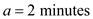Standard deviation of interarrival time,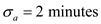Average processing time,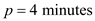Standard deviation of processing time,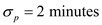Number of servers,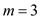a. To find the average waiting time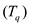, first, compute the coefficients of variation for interarrival and processing time as follows:Compute the utilization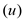as follows:Use the following formula to compute the value of average waiting time.So, the average waiting time for a customer is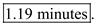b. Use the Little's law to compute the number of emails waiting to be processed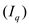.So, the total inventory in the system,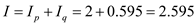So, the number of emails that is delivered and yet to be processed is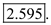Variabilities can affect the overall performance of a process. Any discrepancies in the system should be controlled so as to achieve maximum output. The formula to calculate the length of queue in parallel servers is as shown below: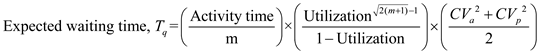Consider the following data for the services provided by a legal services startup: Arrival rate = 10 mails per hour Coefficient of variation CV a = 1 Activity time, p = 5 minutes Standard deviation of service time = 4 minutes a. Calculate the average response time in the queue as shown below: Step 1: Calculate the inter-arrival time by using the formula as shown below:Step 2: Calculate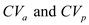to compute the utilization as shown below: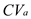= 1 (given in the case)Step 3: Calculate the utilization by using the formula as shown below:Step 4: Calculate the time in queue or total waiting time by using the formula as shown below:Step 5: Calculate the average response time in the queue as shown below:Thus, the average response time is 25.49 minutes. b. Calculate the total number of emails received by the lawyer at the end of the day as shown below:c. Calculate the time that can be dedicated to side by side cases as shown below: Note: The time that can be dedicated to side by side cases will equal to the idle time per day. Calculate the idle time per day as shown below:Thus, the time that can be dedicated to side by side cases is 102 minutes. d. Compute the impact of change in standard deviation of writing the response e-mails as shown below: The idle time is calculated by using the formula,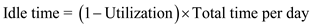The formula for utilization is mentioned below:Where, a is the interarrival time of emails and p is the activity time respectively. One can say that idle time is directly dependent on the utilization but utilization depends on interarrival time of emails and activity time. There is no role of standard deviation in calculating the utilization and idle time. So, there will be no effect of changing the standard deviation on the idle time. e. Calculate the change in average response time if the standard deviation is changed to 0.5 minutes as shown below: Calculate the average response time in the queue as shown below: Step 1: Calculate the inter-arrival time by using the formula as shown below: Standard deviation = 0.5 minutesStep 2: Calculateto compute the utilization as shown below:= 1 (given in the case)Step 3: Calculate the utilization by using the formula as shown below:Step 4: Calculate the time in queue or total waiting time by using the formula as shown below:Step 5: Calculate the average response time in the queue as shown below:Thus, the average response time will be 17.62 minutes.
The demand and supply may vary based on the capacity rate, flow time etc. This results in waiting lines. If the demand exceeds the supply, the waiting time increases. If the supply is more than demand, the utilization decreases. In such cases, the service provider may choose other alternatives to match demand and supply. Following are some of the problems to calculate the utilization, coefficient of variation of inter arrival times, coefficient of variation of processing times and waiting time:…… (1)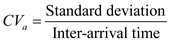…… (2)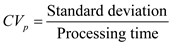…… (3)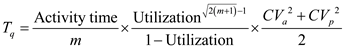…… (4) Consider the following information: Fleet of cars, m is 50 Inter arrival time, a is 2.4 hours Standard deviation is 2.4 hours Average number of days rented, p is 3 days Standard deviation is 1 day The total number of cars in the parking lot are not in use or not utilized. So, first calculate the utilization rate of the cars using equation (1):60% of the cars are utilized. Calculate the cars in parking lot as follows:Therefore, there are 20 cars in the parking lot The average demand will increase to 12 rentals per day if the price is decreased from \$80 by \$25. The following are the changes when there is an increase in demand: Changes in inter arrival time:Changes in processing time:Changes in utilization rate:Change in profit:As there is an increase in the utilization rates and profit, the company should implement the changes in price. Calculate the average wait time as follows: Calculate the coefficient of variation of inter arrival times and coefficient of variation of processing times using equation (2) and equation (3):Calculate the time in queue using equation (4):Hence, the average waiting time is 0.019 hours. The company decides to rent the cars only for 4 days which results in increase of inter arrival time by 3 hours and standard deviation changes to 3 hours. Calculate the average wait time: Calculate the coefficient of variation of inter arrival times and coefficient of variation of processing times as follows:Calculate the time in queue as follows:Hence, the average waiting time is 0.049 hours.